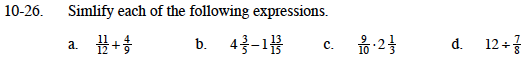### Home > MC1 > Chapter 10 > Lesson 10.1.2 > Problem10-26

10-26.Refer to problem 9-142 for help with this problem.

$1\frac{13}{36}$

Convert these mixed numbers to fractions greater than one before you subtract.

$4\frac{3}{5}=\frac{23}{5} \ \ 1 \frac{13}{15}=\frac{28}{15}$

$\left(\frac{23}{5}\right)\left(\frac{3}{3}\right)=\frac{69}{15}$

$\frac{69}{15}-\frac{28}{15}=\frac{41}{15}$

$\frac{41}{15}=2\frac{11}{15}$

Convert the mixed number to a fraction greater than one and then multiply.

Remember that dividing by a fraction is the same as multiplying by the reciprocal of that fraction.

$\left(\frac{12}{1}\right)\left(\frac{8}{7}\right)=\frac{96}{7}=13\frac{5}{7}$